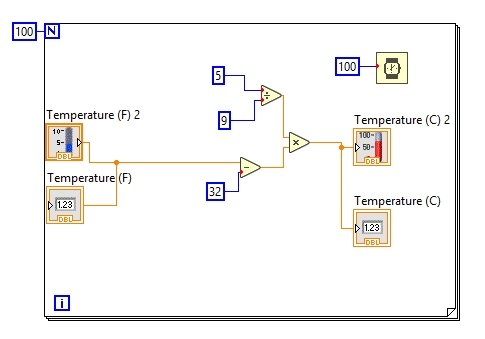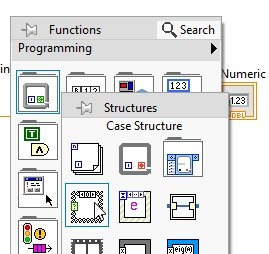# Creating sub VIs in LabView : tutorial 13

In this tutorial you will learn the working of subVI. A subVI is same in LabView as a function or subroutine in text based programming language. At the start you are provided with an introduction to a subVI, including why we need them and what a subVI basically is. After that an explanatory VI is designed in which a subVI is used and some different features of the subVI ar also discussed.

### Introduction to SubVIs in labview

Using sub Vis in LabView is defined as modularity, and by definition, using smaller modules or smaller parts for the overall objective collectively the same tasks as the bigger project will be doing is known as modularity. Creating smaller sections of your overall code is known as a sub VI and in LabView also known as program modularity. In LabView there is no much difference between a VI and a sub VI they are similar to each other. Each sub VI is composed of its own block diagram and its own front panel, but we can only access a sub VI by calling it in its parent VI.

An icon can be seen within the VI representing the sub VI is created when you create a sub VI within a VI and use it. This icon can be customized; the icon for a sub VI is the same as in the upper right corner of a simple Vis block diagram and front panel window. Customizing an icon is also explained in the later section of the tutorial.

If you are a user of C or any other text based programming language you must be familiar with the terms like classes functions and subroutines. The purpose of a subroutine is simply to do a bigger task in smaller portions. Like if you want to build a project using microcontrollers, you can write one function to implement timers’ initializations and other is used to implement the main function, whereas both of them are called in main function.

Translating this in the language of LabView, the timer initialization and main function implementations is done in subVI, however, both of them are called in the main VI of the program. In the following program you will understand the working of sub VIs

### Example of creating SUBVI in labview

• Moving to the programming part, create a VI as we have done in 1st tutorial and save it for future use, as we have done in all previous tutorials.
• To understand the use of sub VIs, what we have to do is to first design a program (bigger one) which we want to convert into modules or sub VIs. To save our time lets use the VI we have already created in the graphs and charts tutorial. The one in which temperature is converted from Fahrenheit to Celsius scale, as shown in the figure below,Figure 1: Block diagram

Figure 2: Creating subVI

Figure 3: Module selection

Figure 4: SubVI created

Figure 5: SubVI front panel

Figure 6: Showing blocking diagram

Figure 7: SubVI block diagram

• From the above figure you can see that this is the same as the blocks we selected the creation part of the subVI.
• One good thing about using subVI is that, we can modify them, to some additional functionality, let’s say in this code we want to modify the conversion. i.e. we want the user to decide whether he want to convert the temperature from Fahrenheit to Celsius or he simply wants to display the temperature as it is.
• This is the same task as we have done before in the case structure tutorial. In this case too, we will use a case structure with a button to decide which case to be used.
• In the subVI place a button on the front panel to toggle the decision of the user. Also from the function palette select a case structure from structures and place all the blocks in that structure as shown below,Figure 8: Case structure placement

Figure 9: Case structure selection

Figure 10: True condition

Figure 11: False condition

Figure 12: properties icon

Figure 13: Selected terminal

Figure 14: Boolean terminal

Figure 15: Boolean terminal on main VI

Figure 16: Complete block diagram

Figure 17: Customizing icon

Exercise:

Design a sub VI that will take two integer inputs and return 4 outputs i.e. their sum, product, quotient and subtracted value.

<<Previous tutorial                                                 Next tutorial>>